# Age-standardized rates

#### 3/9/2022

Age-standardization is used to improve comparison of disease incidence or mortality rates across populations. The term ‘population’ is used to reference any distinct grouping of people, whether it be geographic (e.g., two or more regions), temporal (e.g., two or more years), or social (e.g., two or more genders). Because disease and mortality risk vary strongly by age, we want to control for varying age distributions across groups. Examination of each age-specific rate is indispensable for any analysis, but age-standardized rates provide a single summary index of incidence or mortality risk.

This vignette describes the method of direct age-standardization (Broemeling 2020) and then demonstrates implementation with surveil. The final section extends the methodology to the analysis of health inequality between two groups, where each group is age-stratified (e.g., two racial-ethnic groups in the same region).

## Data

The demonstration will use age-specific cancer incidence for the entire population of the United States of America, 1999-2017.

library(surveil)
data(cancer)
#>   Year   Age Count Population
#> 1 1999    <1   866    3708753
#> 2 1999   1-4  2959   14991152
#> 3 1999   5-9  2226   20146188
#> 4 1999 10-14  2447   19742631
#> 5 1999 15-19  3875   19585857
#> 6 1999 20-24  5969   18148795

We will also use the age distribution of the United States in the year 2000 (2000 U.S. standard million population, see ?standard):

data(standard)
print(standard)
#>    age.id   age standard_pop
#> 1       1    <1        13818
#> 2       2   1-4        55317
#> 3       3   5-9        72533
#> 4       4 10-14        73032
#> 5       5 15-19        72169
#> 6       6 20-24        66478
#> 7       7 25-29        64529
#> 8       8 30-34        71044
#> 9       9 35-39        80762
#> 10     10 40-44        81851
#> 11     11 45-49        72118
#> 12     12 50-54        62716
#> 13     13 55-59        48454
#> 14     14 60-64        38793
#> 15     15 65-69        34264
#> 16     16 70-74        31773
#> 17     17 75-79        26999
#> 18     18 80-84        17842
#> 19     19   85+        15508

Notice that the five-year age groups in the cancer data match the age groups provided by standard.

In some cases, one is only interested in a subset of age groups. For the following examples, we will limit the analysis to persons 40-64 years old:

cancer <- cancer[grep("40-44|45-49|50-54|55-59|60-64", cancer$Age),] standard <- standard[10:14,] head(cancer) #> Year Age Count Population #> 10 1999 40-44 49256 21729686 #> 11 1999 45-49 71420 19235534 #> 12 1999 50-54 99571 16557188 #> 13 1999 55-59 121501 12787366 #> 14 1999 60-64 143968 10435791 #> 29 2000 40-44 50907 22047047 print(standard) #> age.id age standard_pop #> 10 10 40-44 81851 #> 11 11 45-49 72118 #> 12 12 50-54 62716 #> 13 13 55-59 48454 #> 14 14 60-64 38793 If, instead of making this selection, we were to use the entire age distrubtion in our analysis, all of the following discussion and code could proceed unchanged. ## Direct age-standardization ### Methodology Let $$\theta_i$$ be the disease risk in the $$i^{th}$$ age group, and let $$\omega_i$$ be the standard population count for that age group. Then the age-standardized risk is: $SR = \frac{\sum_i \theta_i \omega_i}{\sum_i \omega_i}$ That is, age-standardization consists of multiplying actual age-specific risk levels by false, but fixed, population sizes. ### Crude age-standardized rates To calculate crude age-standardized incidence rates, we use crude age-specific rates $$r_i$$ in place of the (modeled) risk levels $$\theta_i$$: $SR_{\text{crude}} = \frac{\sum_i r_i \omega_i}{\sum_i \omega_i}$ We can calculate age-specific risks $$r_i$$ as follows: standard$Age <- standard$age # make sure names match for the merge cancer_2 <- merge(cancer, standard, by = "Age") # properly join data together cancer_2$crude_rate <- cancer_2$Count / cancer_2$Population  # r_i
cancer_2$crude_reweighted <- cancer_2$crude_rate * cancer_2$standard_pop # r_i * w_i We need the denominator (if we were including all age groups, this would equal one million, by design): M <- sum(standard$standard_pop) # sum(w_i)
M
#>  303932

For 1999, the age-standardized cancer rate per 100,000 at-risk can be calculated as follows:

c2_1999 <- cancer_2[grep(1999, cancer_2$Year),] 100e3 * sum(c2_1999$crude_reweighted) / M  # 100,000 * sum(r_i * w_i) / sum(w_i)
#>  600.802

We can calculate crude age-standardized risk for each year, 1999-2017, as follows:

crude_sr <- aggregate(crude_reweighted ~ Year, data = cancer_2, sum)
crude_sr$SR <- 100e3 * crude_sr$crude_reweighted / M
print(crude_sr)
#>    Year crude_reweighted       SR
#> 1  1999         1826.030 600.8020
#> 2  2000         1852.900 609.6428
#> 3  2001         1869.685 615.1656
#> 4  2002         1866.454 614.1024
#> 5  2003         1828.578 601.6406
#> 6  2004         1828.598 601.6469
#> 7  2005         1830.224 602.1819
#> 8  2006         1848.587 608.2239
#> 9  2007         1872.565 616.1130
#> 10 2008         1865.868 613.9098
#> 11 2009         1864.946 613.6064
#> 12 2010         1807.538 594.7178
#> 13 2011         1813.807 596.7804
#> 14 2012         1779.325 585.4354
#> 15 2013         1776.485 584.5007
#> 16 2014         1783.967 586.9624
#> 17 2015         1787.830 588.2336
#> 18 2016         1767.001 581.3802
#> 19 2017         1734.667 570.7419
plot(crude_sr$Year, crude_sr$SR, type = "l", xlab = "", ylab = 'SR')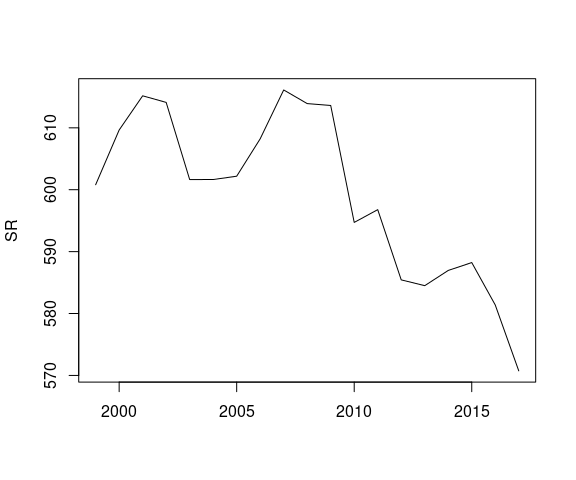## Modeling age-specific risk

For research purposes, we almost always want to base our estimates of age-standardized risk on model-based inferences of age-specific risk $$\theta_i$$, as opposed to crude incidence rates. We model age-specific rates by providing stan_rw with the cancer data and telling it which column contains the time period indicator (Year) and which column contains the grouping variable (Age):

fit <- stan_rw(cancer,
time = Year,
group = Age,
iter = 1500,
chains = 2  #, for speed only; use default chains=4
# cores = 4 # for multi-core processing
)
#> Distribution: normal
#> Distribution: normal
#> Warning: Bulk Effective Samples Size (ESS) is too low, indicating posterior means and medians may be unreliable.
#> Running the chains for more iterations may help. See
#> https://mc-stan.org/misc/warnings.html#bulk-ess
#>  "Setting normal prior(s) for eta_1: "
#>  location scale
#>        -6     5
#>  "\nSetting half-normal prior for sigma: "
#>  location scale
#>         0     1
#>
#> SAMPLING FOR MODEL 'RW' NOW (CHAIN 1).
#> Chain 1:
#> Chain 1: Gradient evaluation took 5.4e-05 seconds
#> Chain 1: 1000 transitions using 10 leapfrog steps per transition would take 0.54 seconds.
#> Chain 1:
#> Chain 1:
#> Chain 1: Iteration:    1 / 1500 [  0%]  (Warmup)
#> Chain 1: Iteration:  751 / 1500 [ 50%]  (Sampling)
#> Chain 1: Iteration: 1500 / 1500 [100%]  (Sampling)
#> Chain 1:
#> Chain 1:  Elapsed Time: 0.676129 seconds (Warm-up)
#> Chain 1:                0.606517 seconds (Sampling)
#> Chain 1:                1.28265 seconds (Total)
#> Chain 1:
#>
#> SAMPLING FOR MODEL 'RW' NOW (CHAIN 2).
#> Chain 2:
#> Chain 2: Gradient evaluation took 2.1e-05 seconds
#> Chain 2: 1000 transitions using 10 leapfrog steps per transition would take 0.21 seconds.
#> Chain 2:
#> Chain 2:
#> Chain 2: Iteration:    1 / 1500 [  0%]  (Warmup)
#> Chain 2: Iteration:  751 / 1500 [ 50%]  (Sampling)
#> Chain 2: Iteration: 1500 / 1500 [100%]  (Sampling)
#> Chain 2:
#> Chain 2:  Elapsed Time: 0.820707 seconds (Warm-up)
#> Chain 2:                0.468544 seconds (Sampling)
#> Chain 2:                1.28925 seconds (Total)
#> Chain 2:

The default plot method will return all of the age-specific cancer risk trends on the same plot; with many age groups and dissimilar risk levels, it is easier to understand the results if, instead, we use a grid of multiple small plots (facet = TRUE) and allow the scale of the y-axes to adjust for each age group (facet_scales = "free"):

plot(fit,
facet = TRUE,          # plot small multiples
facet_scales = "free", # y-axes vary across plots
base_size = 10,      # control text size
size = 0,            # removes crude rates from the plots
scale = 100e3        # plot rates per 100,000
)
#> Plotted rates are per 100,000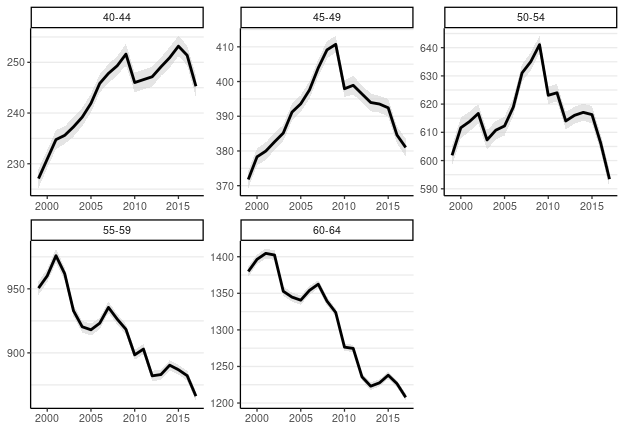The lines indicate estimates of risk $$\theta_i$$ (the means of the posterior probability distributions for $$\theta_i$$) and the shaded intervals correspond to 95% credible intervals for $$\theta_i$$.

In addition to examining trends in age-specific risk (as above), we can also convert each age-specific trend to its annual percent change or cumulative percent change. This time, instead of using the default plotting style, we will use overlaid lines to depict the probability distributions for cumulative percent change:

fit_apc <- apc(fit)
plot(fit_apc,
style = "lines",
base_size = 10,
cum = TRUE)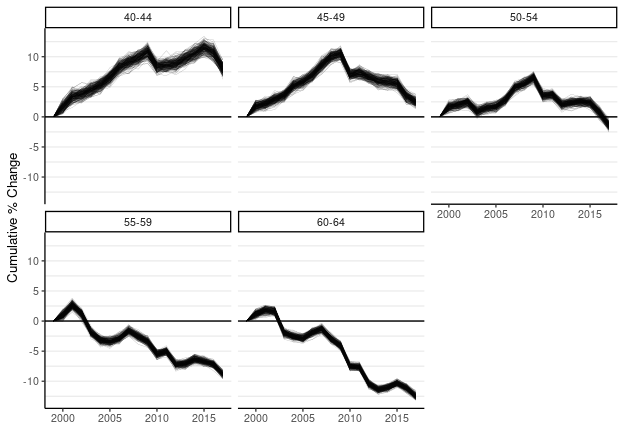Each line drawn on the figure is a draw (sample) from the joint posterior probability distribution for cumulative percent change in $$\theta_i$$: areas of high density of lines have higher probability than areas of low line density; likewise, the general spread of the lines at each time point indicates the range of plausible values.

## Age-standardizing model results

The standardize function takes a fitted model, plus the standard population, and returns standardized rates (SRs):

fit_sr <- standardize(fit,
label = standard$age, standard_pop = standard$standard_pop)

As usual, surveil provides a plotting method for the fit_sr object:

# load ggplot2 to enable additional plot customization
library(ggplot2)
plot(fit_sr, scale = 100e3, base_size = 10) +
labs(title = "US age-standardized cancer incidence per 100,000",
subtitle = "Ages 40-64")
#> Plotted rates are per 100,000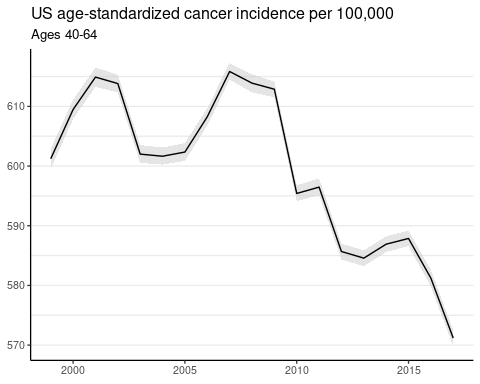as well as a printing method:

print(fit_sr)
#> Summary of age-standardized surveil model results
#> Time periods: 19
#>  time_label stand_rate  .lower  .upper
#>        1999    0.00601 0.00600 0.00603
#>        2000    0.00609 0.00608 0.00611
#>        2001    0.00615 0.00613 0.00617
#>        2002    0.00614 0.00612 0.00615
#>        2003    0.00602 0.00601 0.00604
#>        2004    0.00602 0.00600 0.00603
#>        2005    0.00602 0.00601 0.00604
#>        2006    0.00608 0.00607 0.00610
#>        2007    0.00616 0.00614 0.00617
#>        2008    0.00614 0.00612 0.00615
#>        2009    0.00613 0.00612 0.00614
#>        2010    0.00595 0.00594 0.00597
#>        2011    0.00596 0.00595 0.00598
#>        2012    0.00586 0.00584 0.00587
#>        2013    0.00585 0.00583 0.00586
#>        2014    0.00587 0.00586 0.00588
#>        2015    0.00588 0.00587 0.00589
#>        2016    0.00581 0.00580 0.00583
#>        2017    0.00571 0.00570 0.00572

To learn about the contents of fit_sr, see ?standardize or explores its contents as you would a list (names(fit_sr), fit_sr$standard_summary, head(fit_sr$standard_samples), etc.).

## Comparing age-stratified groups

### Measures of pairwise inequality

What if we are interested in measuring health inequality across two groups, each of which is age stratified?

Let $$\theta_{i,1}$$ be the level of risk in the $$i^{th}$$ age group for the $$1^{st}$$ population, and $$\theta_{i,2}$$ be the risk for the $$i^{th}$$ age group in the $$2^{nd}$$ group; $$P_{i,1}$$ is the age-specific population at risk for group 1. Let group 1 be the group that is expected to have higher risk, and group 2 may be called the ‘reference’ group.

We can compare groups 1 and 2 on three measures of inequality:

1. Standardized rate ratio: $$RR = \frac{SR_1}{SR_2}$$
2. Standardized rate difference: $$RD = SR_1 - SR_2$$
3. Total excess cases: $$EC = \sum_i (\theta_{i,1} - \theta_{i,2}) * P_i = \sum_i RD_i * P_{i,1}$$
4. Total attributable risk: $$AR = \frac{\sum_i RD_i * P_{i,1}}{\sum_i \theta_{i,1} * P_{i,1}}$$

The numerator in the formula for AR contains each age-specific rate difference multiplied by the age-specific population at risk for group 1. If we describe the rate difference as a measure of excess risk, then we can describe the (age-specific) quantity $$RD_i * P_{i,1}$$ as the number of “excess cases,”, i.e., the excess risk expressed in terms of whole cases. The AR formula simply re-expresses excess risk as a fraction of total risk. This is convenient because the standardized RR is based on a fixed and false population distribution, whereas AR is based on the actual population distribution obtaining in reality, each year. Thus, we may often prefer AR over RR as a measure of relative inequality across two age-stratified groups.

Pairwise measures of inequality have an important purpose (tracking health inequalities between historically or currently advantaged and disadvantaged groups), but we should keep in mind the limitations inherent in tracking the progress of any group by comparing that group to a moving target (particularly one that may move in an unfavorable direction). There are rarely only two groups of interest; to measure health inequality across multiple groups, consider Theil’s index (see ?theil and vignette("demonstration")).

### Demonstration

To demonstrate, we will use the same cancer data and create a fake comparison population. This will allow us to avoid introducing a new data set and it will give us control over the results.

Pretend that instead of having cancer incidence data for the entire USA, we have cancer data for two sub-populations, group A and group B. The original cancer data will serve for A (cancer_a), and we will create cancer_b so that its cancer incidence rate is, in relative terms, steadily above that of cancer_a. Group B will have smaller population than group A, and we will also add some extra ‘noise’ to the data.

cancer_a <- cancer
cancer_b <- cancer
## set the case count equal to 0.75 times that of group A
cancer_b$Count <- round(cancer_a$Count * 0.75) +
rpois(n = nrow(cancer), lambda = cancer_a$Count * 0.1) # adds a little noise to the data ## set the population at risk to 0.6 times that of group A cancer_b$Population <- round(cancer_a$Population * 0.6) + rpois(n = nrow(cancer), lambda = cancer_a$Population * 0.1)

Now we can model age-specific risk for Group B (cancer_b):

fit_b <- stan_rw(cancer_b, time = Year, group = Age,
refresh = 0, # silences some printing
iter = 1500,
chains = 2  # for speed only; use default chains=4
# cores = 4 # for multi-core processing
)
#> Distribution: normal
#> Distribution: normal
#>  "Setting normal prior(s) for eta_1: "
#>  location scale
#>        -6     5
#>  "\nSetting half-normal prior for sigma: "
#>  location scale
#>         0     1

And then age-standardize the results:

fit_sr_b <- standardize(fit_b,
label = standard$age, standard_pop = standard$standard_pop)

Finally, we place the two age-standardized models into a list (preferably a named list), and pass the list to the group_diff function (see ?group_diff for more details):

fit_sr_list <- list(B = fit_sr_b, A = fit_sr)
ineq <- group_diff(fit_sr_list)
plot(ineq, base_size = 10)
#> Rate differences (RD) are per 100,000 at risk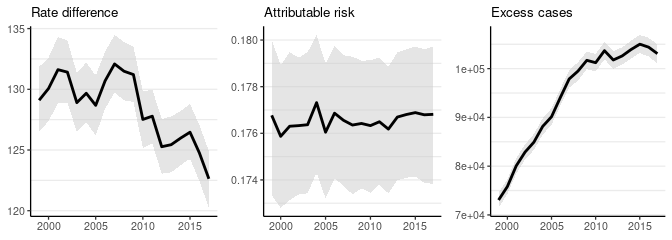We see that relative inequality (AR) is about constant (as expected), the rate difference falls (because the total level of cancer risk has fallen over time), and excess cases increase over time (because the total population at risk is both growing and aging).

print(ineq)
#> Summary of Pairwise Inequality
#> Target group: B
#> Reference group: A
#> Time periods observed: 19
#> Cumulative excess cases (EC): 1,793,853 [1785643, 1801873]
#> Cumulative EC as a fraction of group risk (PAR): 0.18 [0.18, 0.18]
#>  time   Rate     RD  PAR  RR     EC
#>  1999 0.0073 0.0013 0.18 1.2  73056
#>  2000 0.0074 0.0013 0.18 1.2  75820
#>  2001 0.0075 0.0013 0.18 1.2  80059
#>  2002 0.0075 0.0013 0.18 1.2  82888
#>  2003 0.0073 0.0013 0.18 1.2  84910
#>  2004 0.0073 0.0013 0.18 1.2  88157
#>  2005 0.0073 0.0013 0.18 1.2  90129
#>  2006 0.0074 0.0013 0.18 1.2  94085
#>  2007 0.0075 0.0013 0.18 1.2  97881
#>  2008 0.0075 0.0013 0.18 1.2  99498
#>  2009 0.0074 0.0013 0.18 1.2 101701
#>  2010 0.0072 0.0013 0.18 1.2 101220
#>  2011 0.0072 0.0013 0.18 1.2 103684
#>  2012 0.0071 0.0013 0.18 1.2 101778
#>  2013 0.0071 0.0013 0.18 1.2 102597
#>  2014 0.0071 0.0013 0.18 1.2 103904
#>  2015 0.0071 0.0013 0.18 1.2 105008
#>  2016 0.0071 0.0012 0.18 1.2 104407
#>  2017 0.0069 0.0012 0.18 1.2 103071